This is “Adding and Subtracting Rational Expressions”, section 7.3 from the book Beginning Algebra (v. 1.0). For details on it (including licensing), click here.

Has this book helped you? Consider passing it on:
Creative Commons supports free culture from music to education. Their licenses helped make this book available to you.
DonorsChoose.org helps people like you help teachers fund their classroom projects, from art supplies to books to calculators.

## 7.3 Adding and Subtracting Rational Expressions

### Learning Objectives

1. Add and subtract rational expressions with common denominators.
2. Add and subtract rational expressions with unlike denominators.
3. Add and subtract rational functions.

## Adding and Subtracting with Common Denominators

Adding and subtracting rational expressions is similar to adding and subtracting fractions. Recall that if the denominators are the same, we can add or subtract the numerators and write the result over the common denominator.When working with rational expressions, the common denominator will be a polynomial. In general, given polynomials P, Q, and R, where $Q≠0$, we have the following:In this section, assume that all variable factors in the denominator are nonzero.

Example 1: Add: $3y+7y$.

Solution: Add the numerators 3 and 7, and write the result over the common denominator, y.Answer: $10y$

Example 2: Subtract: $x−52x−1−12x−1$.

Solution: Subtract the numerators $x−5$ and 1, and write the result over the common denominator, $2x−1$.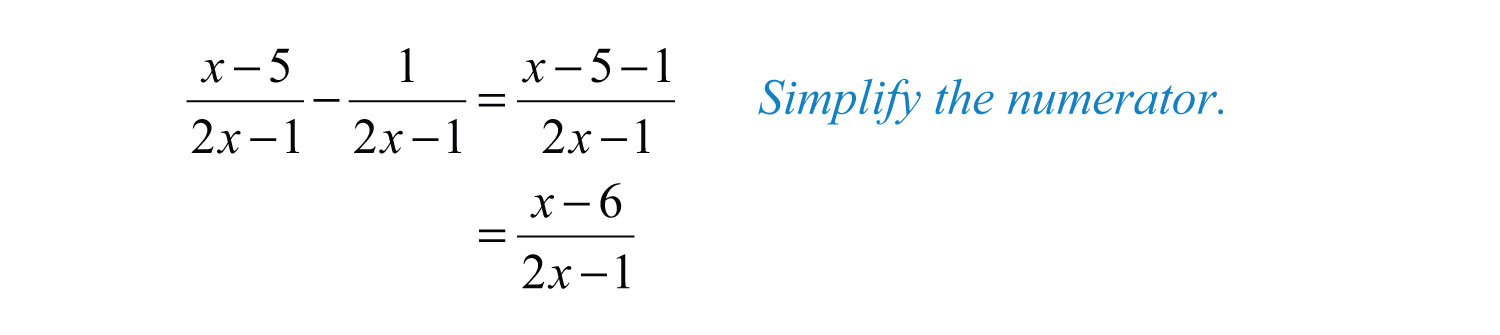Answer: $x−62x−1$

Example 3: Subtract: $2x+7(x+5)(x−3)−x+10(x+5)(x−3)$.

Solution: We use parentheses to remind us to subtract the entire numerator of the second rational expression.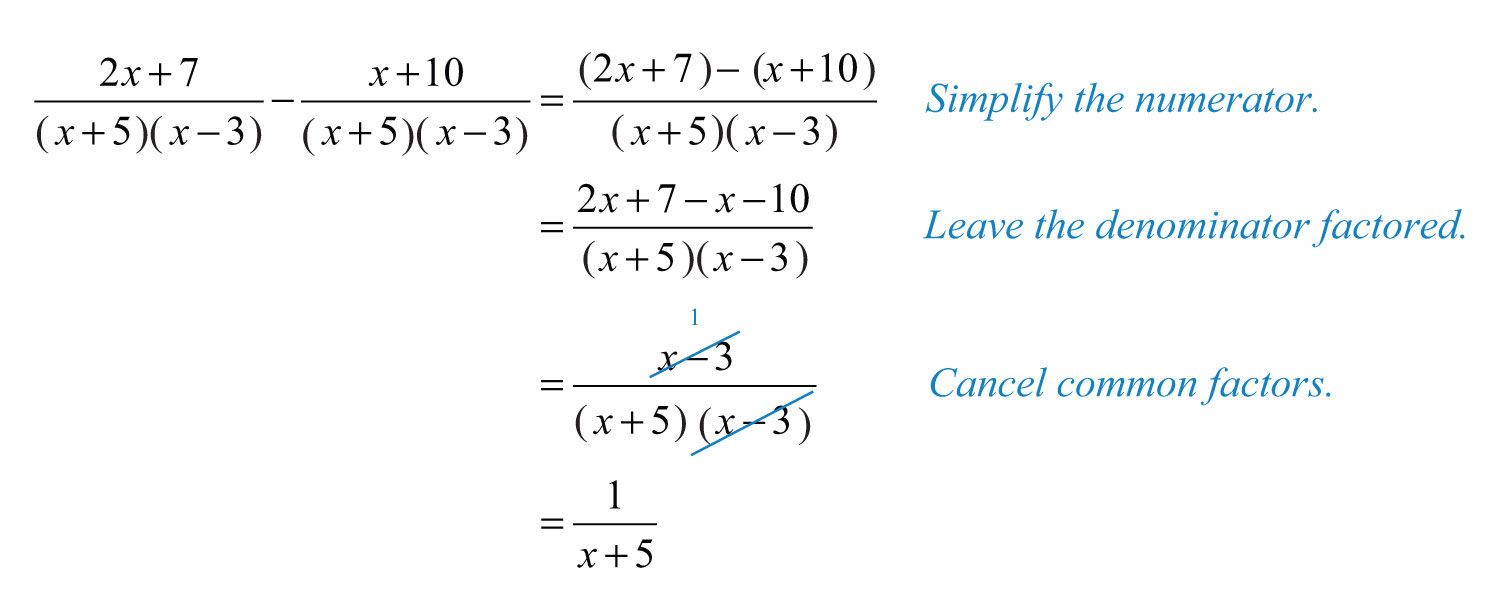Answer: $1x+5$

Example 4: Simplify: $2x2+10x+3x2−36−x2+6x+5x2−36+x−4x2−36$.

Solution: Subtract and add the numerators. Make use of parentheses and write the result over the common denominator, $x2−36$.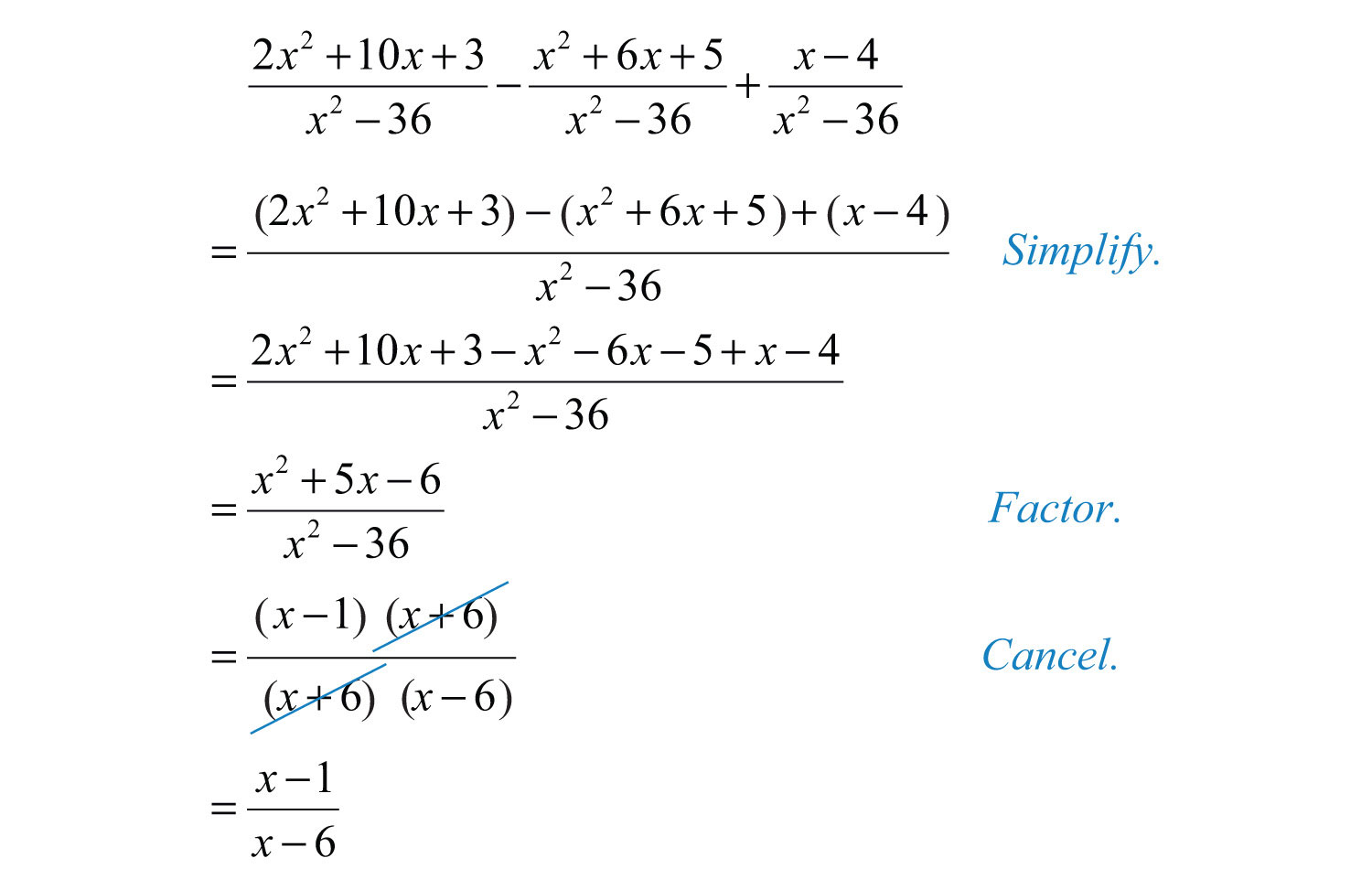Answer: $x−1x−6$

Try this! Subtract: $x2+12x2−7x−4−x2−2x2x2−7x−4$.

Answer: $1x−4$

### Video Solution

(click to see video)

## Adding and Subtracting with Unlike Denominators

To add rational expressions with unlike denominators, first find equivalent expressions with common denominators. Do this just as you have with fractions. If the denominators of fractions are relatively prime, then the least common denominator (LCD) is their product. For example,Multiply each fraction by the appropriate form of 1 to obtain equivalent fractions with a common denominator.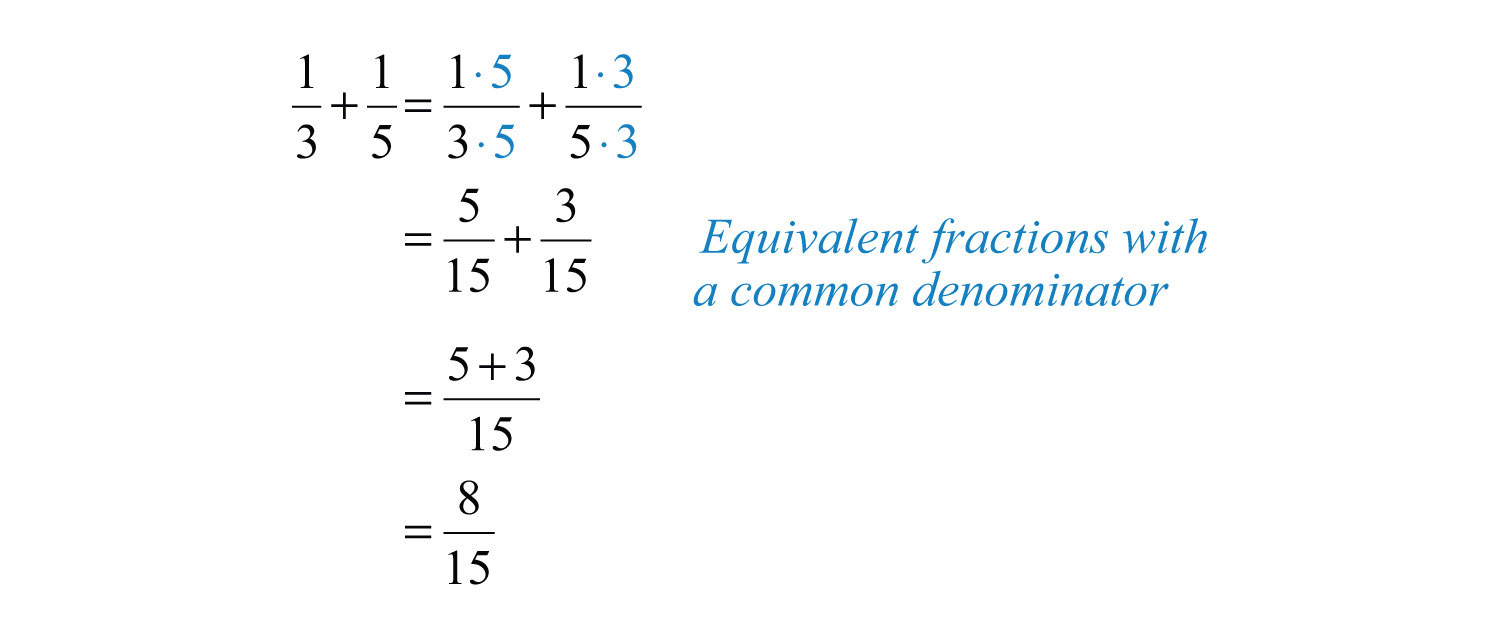The process of adding and subtracting rational expressions is similar. In general, given polynomials P, Q, R, and S, where $Q≠0$ and $S≠0$, we have the following:In this section, assume that all variable factors in the denominator are nonzero.

Example 5: Add: $1x+1y$.

Solution: In this example, the $LCD=xy$. To obtain equivalent terms with this common denominator, multiply the first term by $yy$ and the second term by $xx$.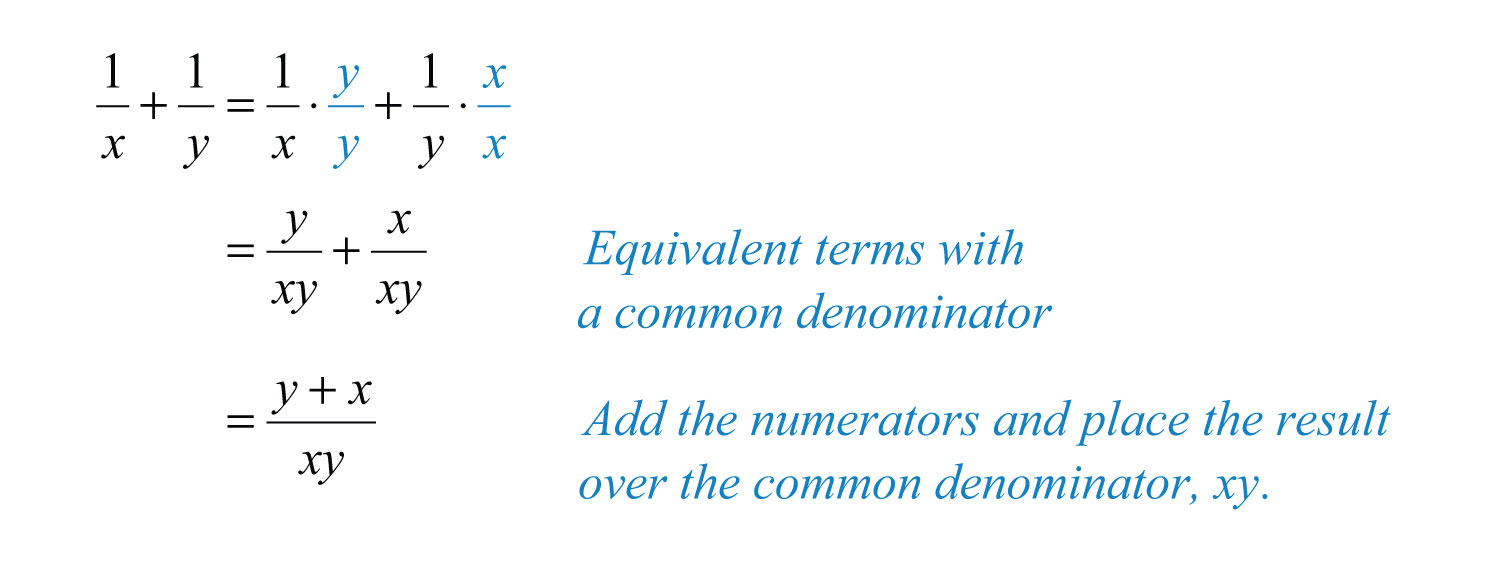Answer: $y+xxy$

Example 6: Subtract: $1y−1y−3$.

Solution: Since the $LCD=y(y−3)$, multiply the first term by 1 in the form of $(y−3)(y−3)$ and the second term by $yy$.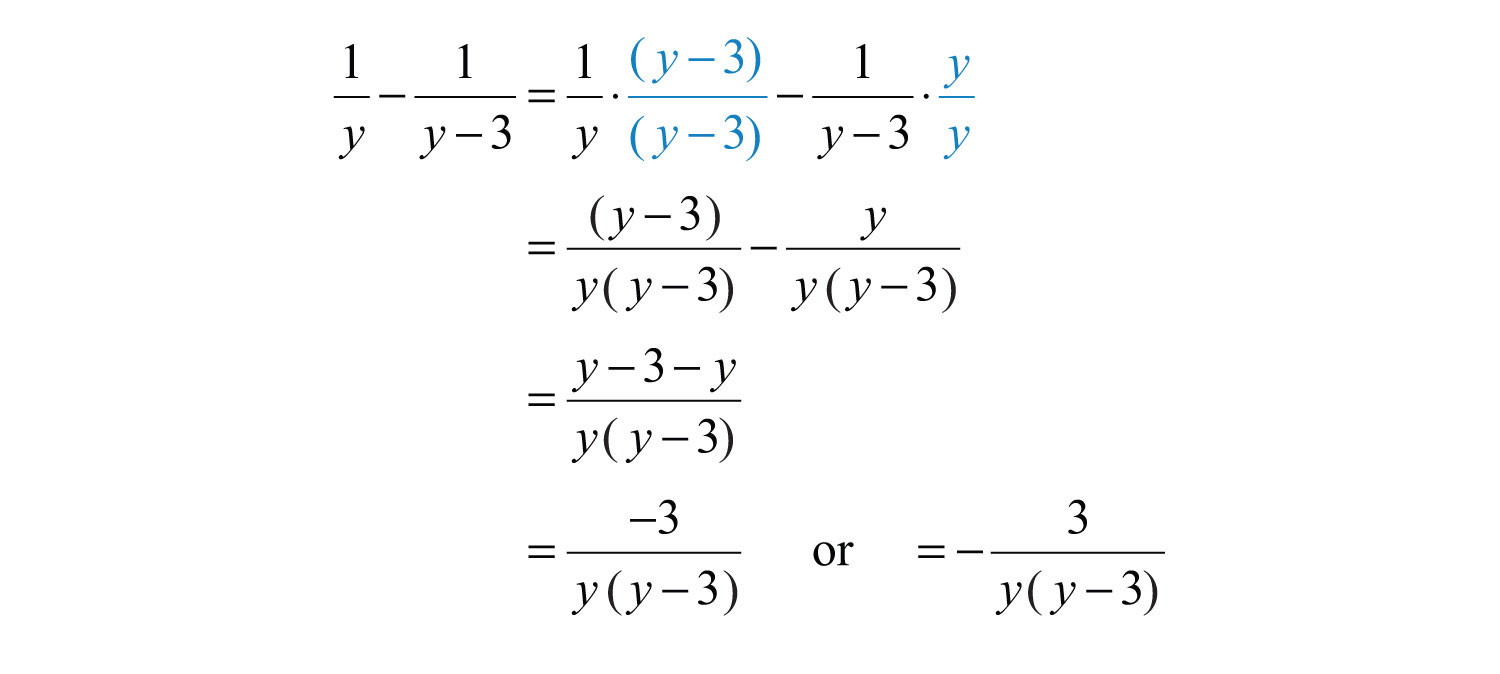Answer: $−3y(y−3)$

It is not always the case that the LCD is the product of the given denominators. Typically, the denominators are not relatively prime; thus determining the LCD requires some thought. Begin by factoring all denominators. The LCD is the product of all factors with the highest power. For example, giventhere are three base factors in the denominator: $x$, $(x+2)$, and $(x−3)$. The highest powers of these factors are $x3$, $(x+2)2$, and $(x−3)1$. Therefore,The general steps for adding or subtracting rational expressions are illustrated in the following example.

Example 7: Subtract: $xx2+4x+3−3x2−4x−5$.

Solution:

Step 1: Factor all denominators to determine the LCD.The $LCD is (x+1)(x+3)(x−5)$.

Step 2: Multiply by the appropriate factors to obtain equivalent terms with a common denominator. To do this, multiply the first term by $(x−5)(x−5)$ and the second term by $(x+3)(x+3)$.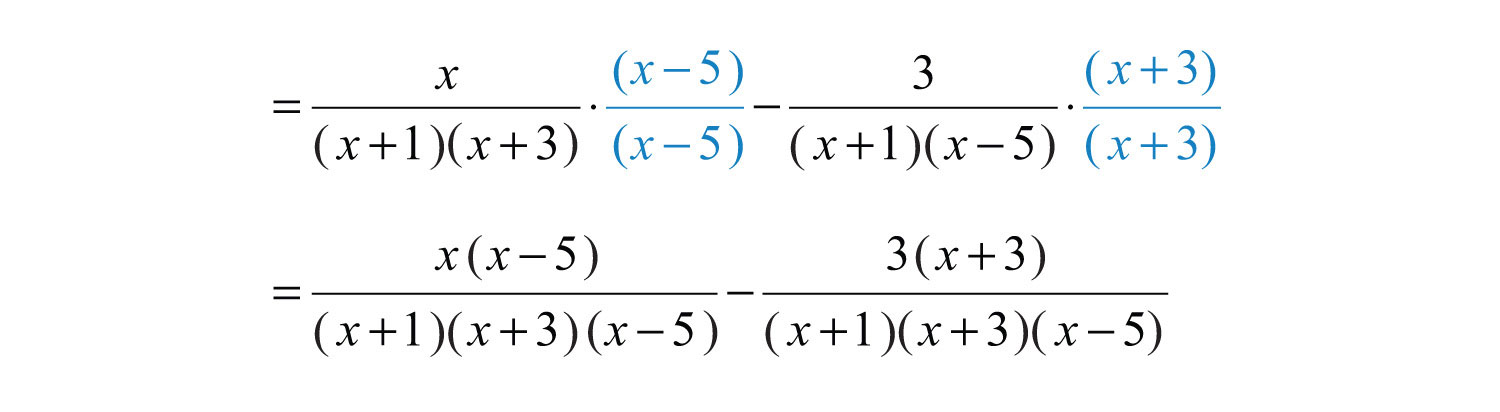Step 3: Add or subtract the numerators and place the result over the common denominator.Step 4: Simplify the resulting algebraic fraction.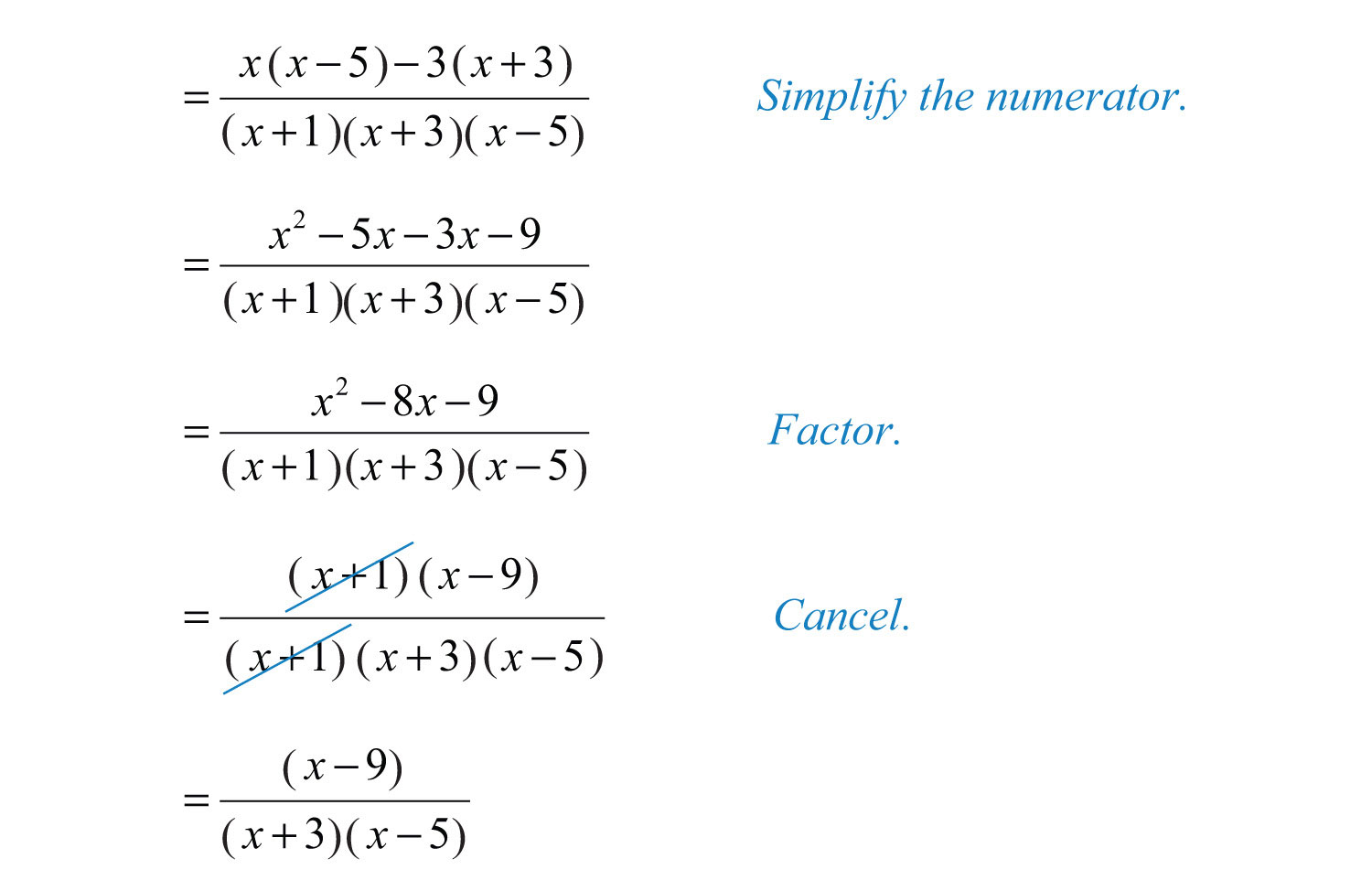Answer: $(x−9)(x+3)(x−5)$

Example 8: Subtract: $x2−9x+18x2−13x+36−xx−4$.

Solution: It is best not to factor the numerator, $x2−9x+18$, because we will most likely need to simplify after we subtract.Answer: $18(x−4)(x−9)$

Example 9: Subtract: $1x2−4−12−x$.

Solution: First, factor the denominators and determine the LCD. Notice how the opposite binomial property is applied to obtain a more workable denominator.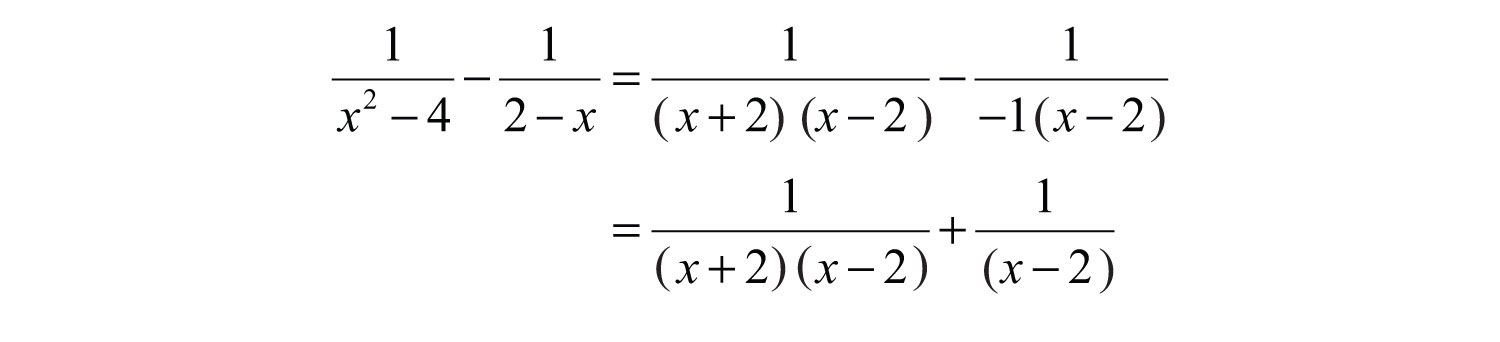The LCD is $(x+2)(x−2)$. Multiply the second term by 1 in the form of $(x+2)(x+2)$.Now that we have equivalent terms with a common denominator, add the numerators and write the result over the common denominator.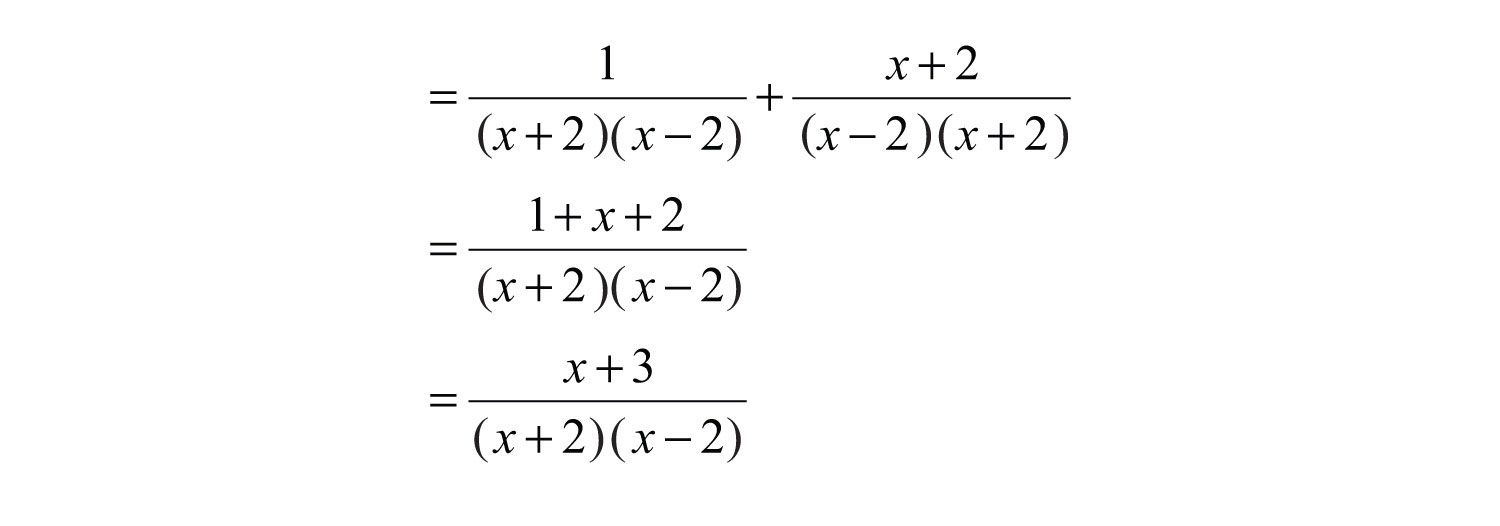Answer: $x+3(x+2)(x−2)$

Example 10: Simplify: $y−1y+1−y+1y−1+y2−5y2−1$.

Solution: Begin by factoring the denominator.We can see that the . Find equivalent fractions with this denominator.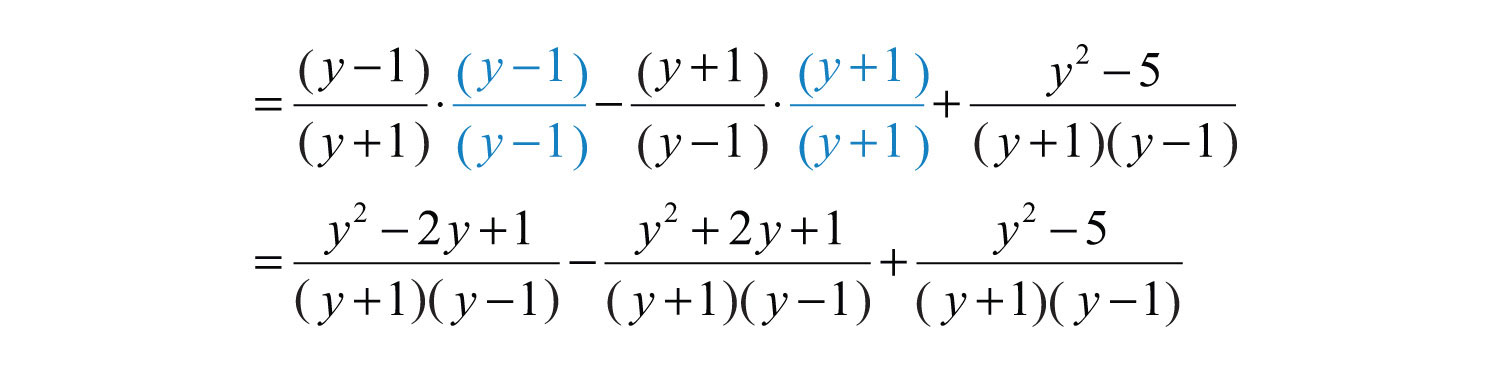Next, subtract and add the numerators and place the result over the common denominator.Finish by simplifying the resulting rational expression.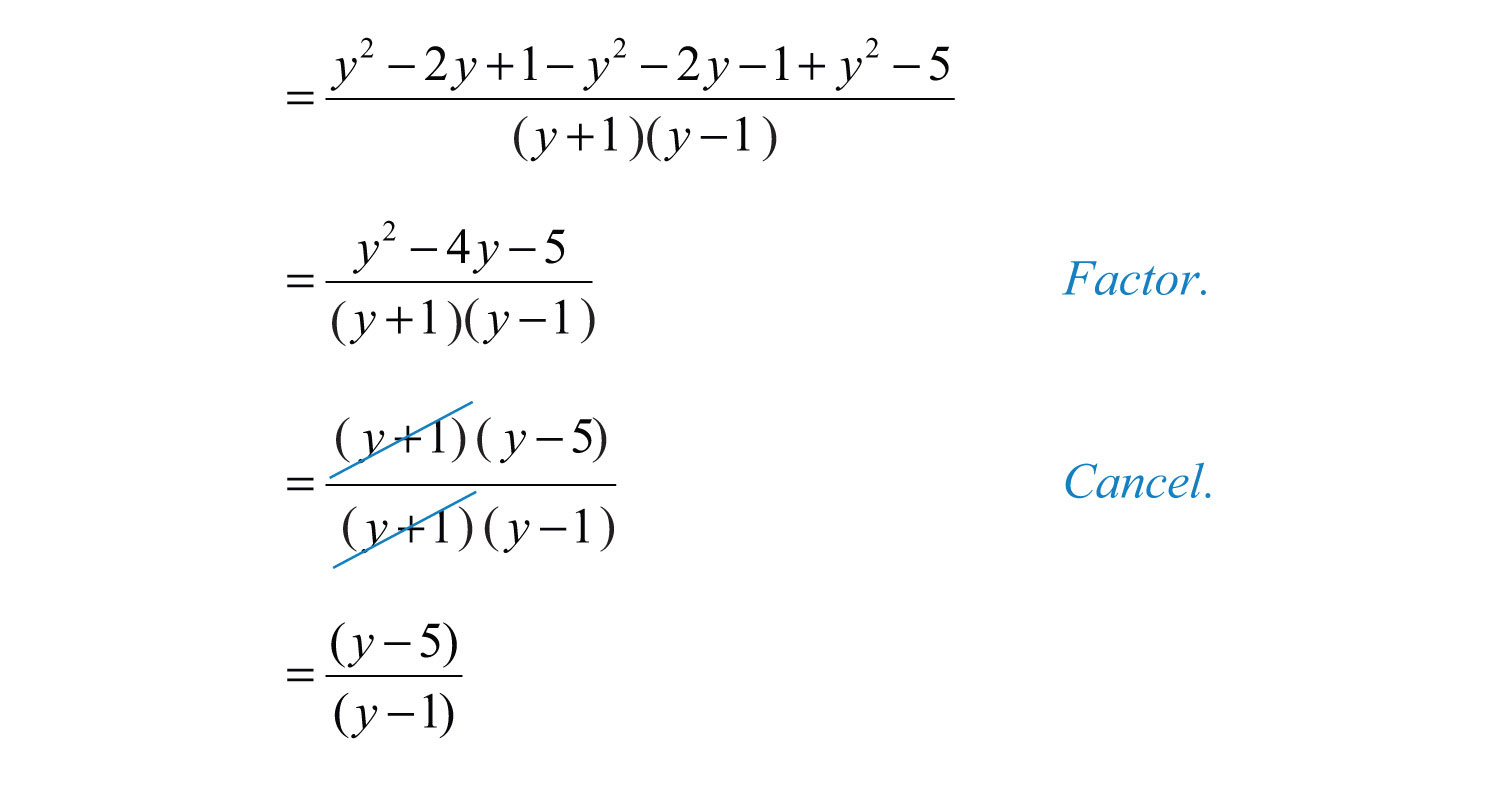Answer: $y−5y−1$

Try this! Simplify: $−2x2−1+x1+x−51−x$.

Answer: $x+3x−1$

### Video Solution

(click to see video)

Rational expressions are sometimes expressed using negative exponents. In this case, apply the rules for negative exponents before simplifying the expression.

Example 11: Simplify: $y−2+(y−1)−1$.

Solution: Recall that $x−n=1xn$. We begin by rewriting the negative exponents as rational expressions.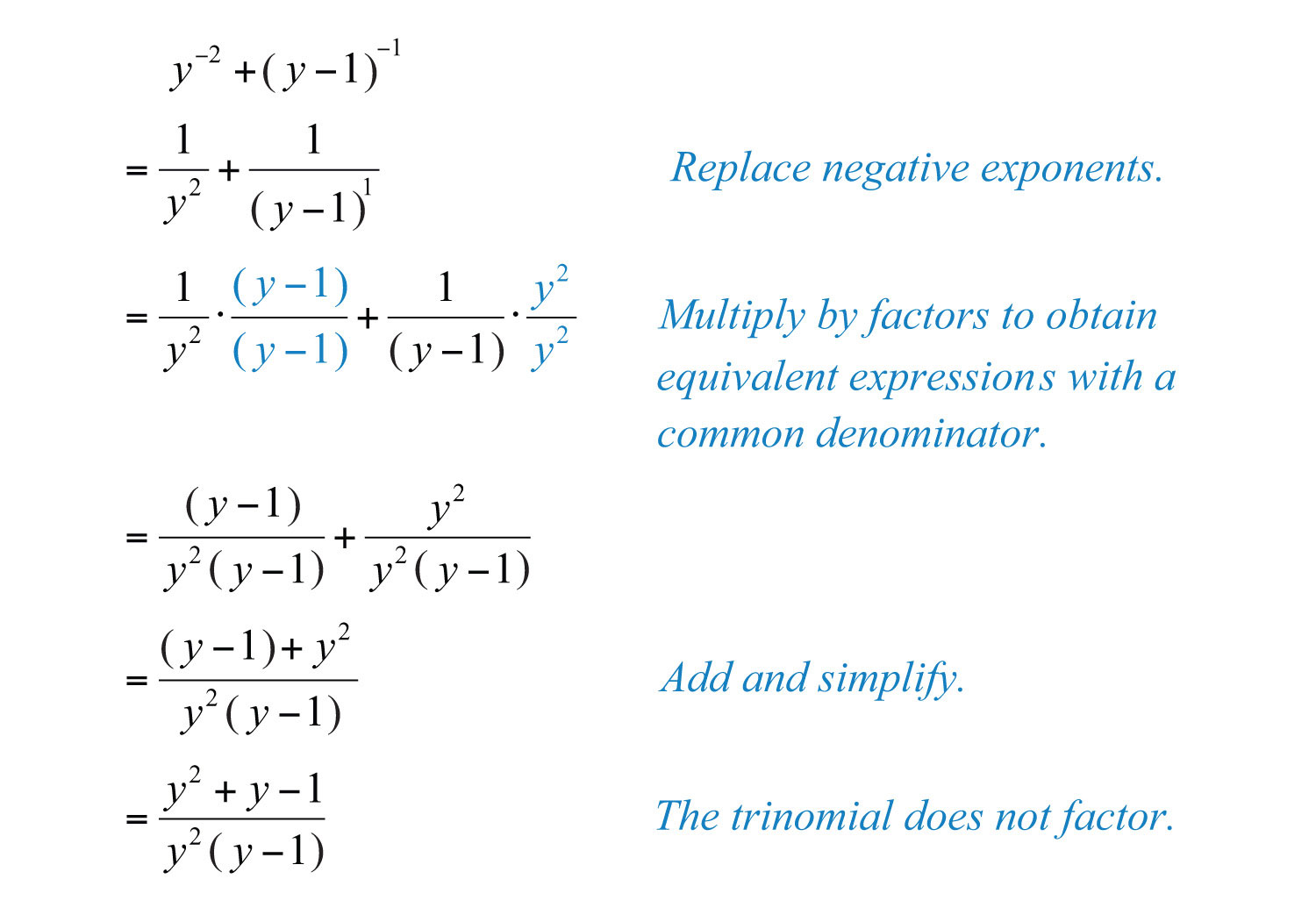Answer: $y2+y−1y2(y−1)$

## Adding and Subtracting Rational Functions

We can simplify sums or differences of rational functions using the techniques learned in this section. The restrictions of the result consist of the restrictions to the domains of each function.

Example 12: Calculate $(f+g)(x)$, given $f(x)=1x+3$ and $g(x)=1x−2$, and state the restrictions.

Solution: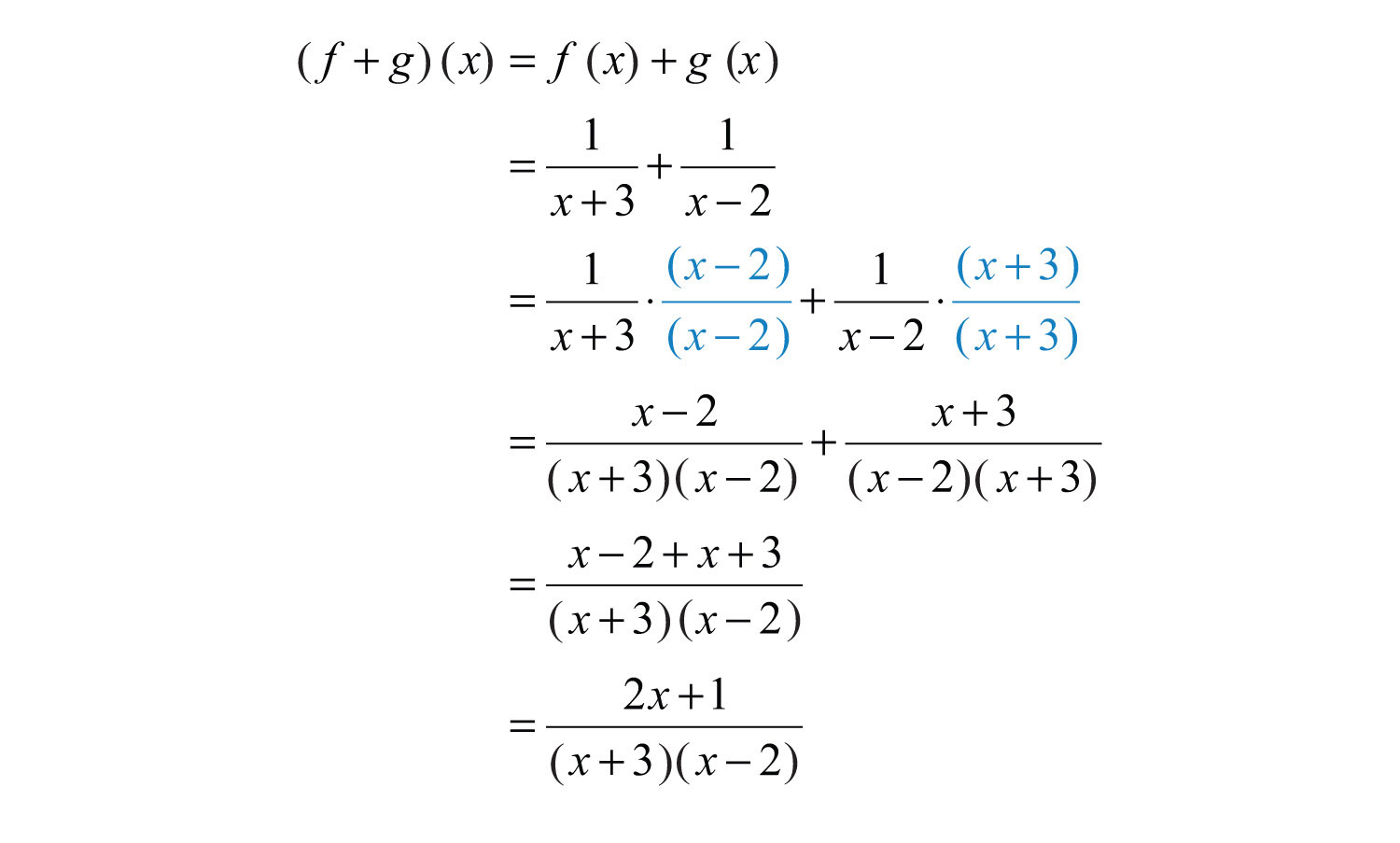Here the domain of f consists of all real numbers except −3, and the domain of g consists of all real numbers except 2. Therefore, the domain of f + g consists of all real numbers except −3 and 2.

Answer: $2x+1(x+3)(x−2)$, where $x≠−3, 2$

Example 13: Calculate $(f−g)(x)$, given $f(x)=x(x−1)x2−25$ and $g(x)=x−3x−5$, and state the restrictions to the domain.

Solution: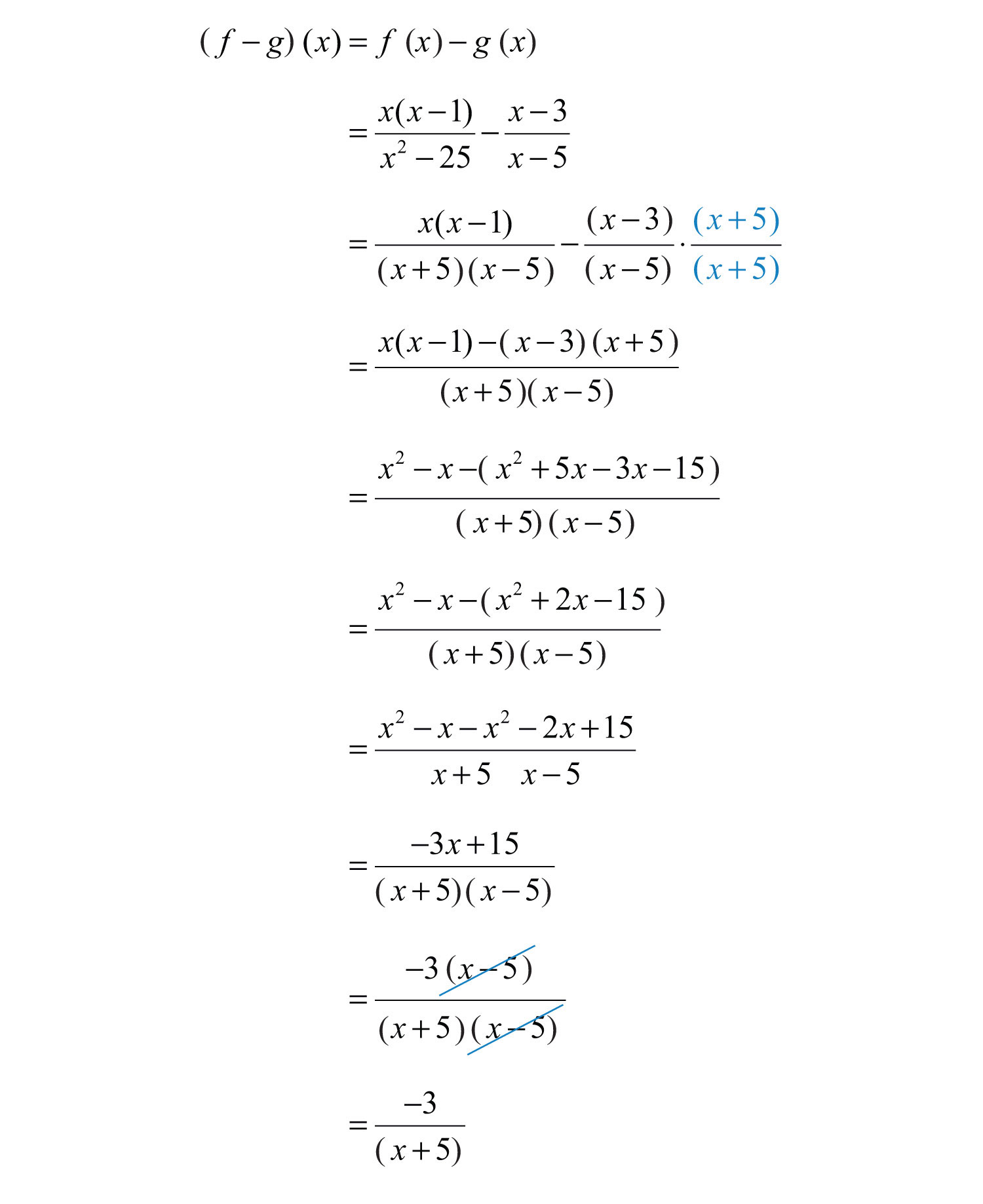The domain of f consists of all real numbers except 5 and −5, and the domain of g consists of all real numbers except 5. Therefore, the domain of fg consists of all real numbers except −5 and 5.

Answer: $−3x+5$, where $x≠±5$

### Key Takeaways

• When adding or subtracting rational expressions with a common denominator, add or subtract the expressions in the numerator and write the result over the common denominator.
• To find equivalent rational expressions with a common denominator, first factor all denominators and determine the least common multiple. Then multiply numerator and denominator of each term by the appropriate factor to obtain a common denominator. Finally, add or subtract the expressions in the numerator and write the result over the common denominator.
• The restrictions to the domain of a sum or difference of rational functions consist of the restrictions to the domains of each function.

### Topic Exercises

Part A: Adding and Subtracting with Common Denominators

Simplify. (Assume all denominators are nonzero.)

1. $3x+7x$

2. $9x−10x$

3. $xy−3y$

4. $4x−3+6x−3$

5. $72x−1−x2x−1$

6. $83x−8−3x3x−8$

7. $2x−9+x−11x−9$

8. $y+22y+3−y+32y+3$

9. $2x−34x−1−x−44x−1$

10. $2xx−1−3x+4x−1+x−2x−1$

11. $13y−2y−93y−13−5y3y$

12. $−3y+25y−10+y+75y−10−3y+45y−10$

13. $x(x+1)(x−3)−3(x+1)(x−3)$

14. $3x+5(2x−1)(x−6)−x+6(2x−1)(x−6)$

15. $xx2−36+6x2−36$

16. $xx2−81−9x2−81$

17. $x2+2x2+3x−28+x−22x2+3x−28$

18. $x2x2−x−3−3−x2x2−x−3$

Part B: Adding and Subtracting with Unlike Denominators

Simplify. (Assume all denominators are nonzero.)

19. $12+13x$

20. $15x2−1x$

21. $112y2+310y3$

22. $1x−12y$

23. $1y−2$

24. $3y+2−4$

25. $2x+4+2$

26. $2y−1y2$

27. $3x+1+1x$

28. $1x−1−2x$

29. $1x−3+1x+5$

30. $1x+2−1x−3$

31. $xx+1−2x−2$

32. $2x−3x+5−xx−3$

33. $y+1y−1+y−1y+1$

34. $3y−13y−y+4y−2$

35. $2x−52x+5−2x+52x−5$

36. $22x−1−2x+11−2x$

37. $3x+4x−8−28−x$

38. $1y−1+11−y$

39. $2x2x2−9+x+159−x2$

40. $xx+3+1x−3−15−x(x+3)(x−3)$

41. $2x3x−1−13x+1+2(x−1)(3x−1)(3x+1)$

42. $4x2x+1−xx−5+16x−3(2x+1)(x−5)$

43. $x3x+2x−2+43x(x−2)$

44. $−2xx+6−3x6−x−18(x−2)(x+6)(x−6)$

45. $xx+5−1x−7−25−7x(x+5)(x−7)$

46. $xx2−2x−3+2x−3$

47. $1x+5−x2x2−25$

48. $5x−2x2−4−2x−2$

49. $1x+1−6x−3x2−7x−8$

50. $3x9x2−16−13x+4$

51. $2xx2−1+1x2+x$

52. $x(4x−1)2x2+7x−4−x4+x$

53. $3x23x2+5x−2−2x3x−1$

54. $2xx−4−11x+4x2−2x−8$

55. $x2x+1+6x−242x2−7x−4$

56. $1x2−x−6+1x2−3x−10$

57. $xx2+4x+3−3x2−4x−5$

58. $y+12y2+5y−3−y4y2−1$

59. $y−1y2−25−2y2−10y+25$

60. $3x2+24x2−2x−8−12x−4$

61. $4x2+28x2−6x−7−28x−7$

62. $a4−a+a2−9a+18a2−13a+36$

63. $3a−12a2−8a+16−a+24−a$

64. $a2−142a2−7a−4−51+2a$

65. $1x+3−xx2−6x+9+3x2−9$

66. $3xx+7−2xx−2+23x−10x2+5x−14$

67. $x+3x−1+x−1x+2−x(x+11)x2+x−2$

68. $−2x3x+1−4x−2+4(x+5)3x2−5x−2$

69. $x−14x−1−x+32x+3−3(x+5)8x2+10x−3$

70. $3x2x−3−22x+3−6x2−5x−94x2−9$

71. $1y+1+1y+2y2−1$

72. $1y−1y+1+1y−1$

73. $5−2+2−1$

74. $6−1+4−2$

75. $x−1+y−1$

76. $x−2−y−1$

77. $(2x−1)−1−x−2$

78. $(x−4)−1−(x+1)−1$

79. $3x2(x−1)−1−2x$

80. $2(y−1)−2−(y−1)−1$

Part C: Adding and Subtracting Rational Functions

Calculate $(f+g)(x)$ and $(f−g)(x)$ and state the restrictions to the domain.

81. $f(x)=13x$ and $g(x)=1x−2$

82. $f(x)=1x−1$ and $g(x)=1x+5$

83. $f(x)=xx−4$ and $g(x)=14−x$

84. $f(x)=xx−5$ and $g(x)=12x−3$

85. $f(x)=x−1x2−4$ and $g(x)=4x2−6x−16$

86. $f(x)=5x+2$ and $g(x)=3x+4$

Calculate $(f+f)(x)$ and state the restrictions to the domain.

87. $f(x)=1x$

88. $f(x)=12x$

89. $f(x)=x2x−1$

90. $f(x)=1x+2$

Part D: Discussion Board

91. Explain to a classmate why this is incorrect: $1x2+2x2=32x2$.

92. Explain to a classmate how to find the common denominator when adding algebraic expressions. Give an example.

1: $10x$

3: $x−3y$

5: $7−x2x−1$

7: 1

9: $x+14x−1$

11: $y−1y$

13: $1x+1$

15: $1x−6$

17: $x+5x+7$

19: $3x+26x$

21: $5y+1860y3$

23: $1−2yy$

25: $2(x+5)x+4$

27: $4x+1x(x+1)$

29: $2(x+1)(x−3)(x+5)$

31: $x2−4x−2(x−2)(x+1)$

33: $2(y2+1)(y+1)(y−1)$

35: $−40x(2x+5)(2x−5)$

37: $3(x+2)x−8$

39: $2x+5x+3$

41: $2x+13x+1$

43: $x2+4x+43x(x−2)$

45: $x−6x−7$

47: $−x2+x−5(x+5)(x−5)$

49: $−5x−8$

51: $2x−1x(x−1)$

53: $x(x−4)(x+2)(3x−1)$

55: $x+62x+1$

57: $x−9(x−5)(x+3)$

59: $y2−8y−5(y+5)(y−5)2$

61: $4xx+1$

63: $a+5a−4$

65: $−6x(x+3)(x−3)2$

67: $x−7x+2$

69: $−x−54x−1$

71: $2y−1y(y−1)$

73: $2750$

75: $x+yxy$

77: $(x−1)2x2(2x−1)$

79: $x(x+2)x−1$

81: $(f+g)(x)=2(2x−1)3x(x−2)$; $(f−g)(x)=−2(x+1)3x(x−2)$; $x≠0, 2$

83: $(f+g)(x)=x−1x−4$; $(f−g)(x)=x+1x−4$; $x≠4$

85: $(f+g)(x)=x(x−5)(x+2)(x−2)(x−8)$; $(f−g)(x)=x2−13x+16(x+2)(x−2)(x−8)$; $x≠−2, 2, 8$

87: $(f+f)(x)=2x$; $x≠0$

89: $(f+f)(x)=2x2x−1$; $x≠12$